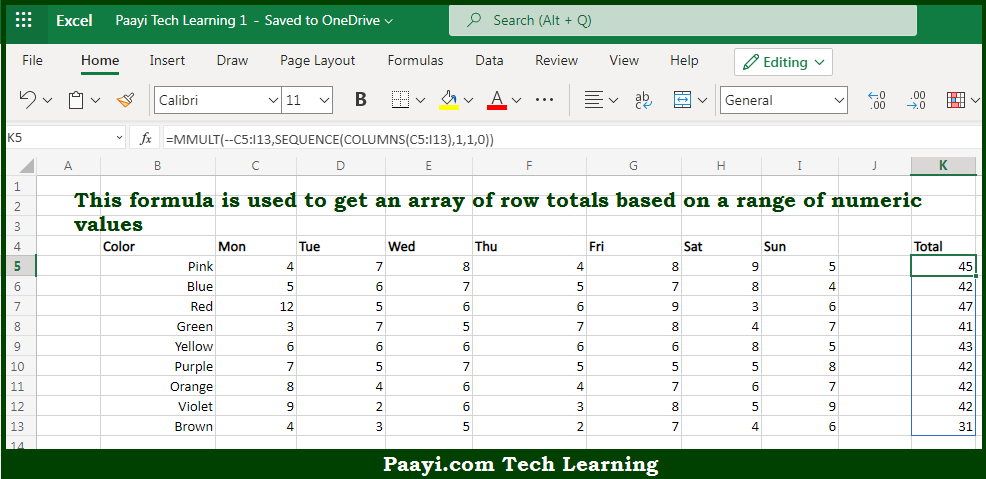# Learn How to Get Row Totals in Microsoft Excel

Written by | 0 Comments | 479 Views

In this article, you will learn how to evaluate things in Dynamic Arrays with formulas in Microsoft Excel using a single/combination(s) of functions. You will also know How to Get Row Totals and see the generic formula.

Learn How to Get Row Totals in Microsoft Excel

The main purpose of this formula is to get an array of row totals based on a range of numeric values. Here we will learn how to get row totals in the given data range in the workbook in Microsoft Excel. That implies, with the help of a formula based on the MMULT, SEQUENCE and COLUMNS function you can able to get an array of row totals based on a range of numeric values. So, with the help of this formula, you can able to get row totals in the given data range in the workbook in Microsoft Excel.

General Formula to Get Row Totals

=MMULT(--rng,SEQUENCE(COLUMNS(rng),1,1,0))

The Explanation for to Get Row TotalsSo we know that with the help of the given formula above you can able to get an array of row totals based on a range of numeric values. Here we will learn how to get row totals in the given data range in the workbook in Microsoft Excel. As we know that there are some situations where you want an array of sums instead of a single sum. Functions like SUM won't work, as they aggregate results and returns a single value. The MMULT function is used to return the matrix product of two arrays. It is also called the "dot product" is useful in these cases. So, with the help of this formula, you can able to get an array of row totals based on a range of numeric values. Here we will learn how to get row totals in the given data range in the workbook in Microsoft Excel.